Skaits: 0

Kopā: 0,00

# Cube

### Cube

This animation demonstrates the components (vertices, edges, diagonals and faces) of the cube, one of the Platonic solids.

Matemātika

Atslēgvārdi

kubs, virsotne, malu, seja, sejas diagonāle, telpa pa diagonāli, kaimiņu sejas, cietā ģeometrija, Matemātika

### Saistītie vienumi

Informācija Colouring a cube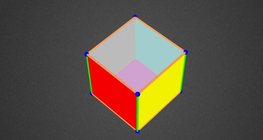#### Colouring a cube

Colouring the vertices, edges and faces of a given cube according to the criteria specified in the exercise.

Informācija Cube (exercises)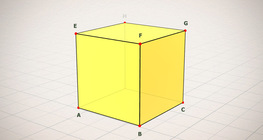#### Cube (exercises)

Edges, diagonals and faces of a cube can be identified by its vertices.

Informācija Cube of cubes#### Cube of cubes

An exercise about the regular hexahedron built from unit cubes to help deepen your knowledge of cubes.

Informācija Net of a cube (exercises)#### Net of a cube (exercises)

Not all nets consisting of 6 congruent squares are foldable into cubes.

Informācija Building shapes (one colour)#### Building shapes (one colour)

Build 3D shapes from unit cubes with the help of several views.

Informācija Cube sections (exercise)#### Cube sections (exercise)

Examining solids formed by the intersection of a cube and a plane.

Informācija Cuboid#### Cuboid

A cuboid is a polyhedron with six rectangular faces.

Informācija Dividing Space into Regions by 3 Planes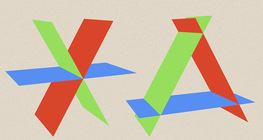#### Dividing Space into Regions by 3 Planes

Division of space by three intersecting planes in several different arrangements.

Informācija Geometric transformations – translation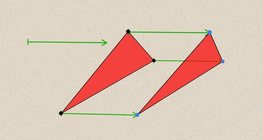#### Geometric transformations – translation

This animation demonstrates geometric translation in both plane and space.

Informācija Grouping of solids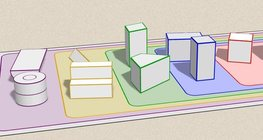#### Grouping of solids

This animation demonstrates various groups of solids through examples.

Informācija Grouping of solids 1#### Grouping of solids 1

This animation demonstrates various groups of solids through examples.

Informācija Nets of a cuboid (exercises)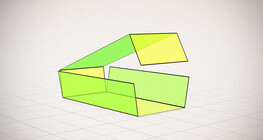#### Nets of a cuboid (exercises)

This animation demonstrates the different nets of a cuboid and includes a game.

Informācija Perimetrs, laukums, virsmas laukums un tilpums#### Perimetrs, laukums, virsmas laukums un tilpums

Šajā animācijā iepazīstināsim ar formulām, kas ļauj aprēķināt figūru perimetru un laukumu, kā arī ģeometrisku ķermeņu virsmas laukumu un tilpumu.

Informācija Platonic solids#### Platonic solids

This animation demonstrates the five regular three-dimensional (or Platonic) solids, the best known of which is the cube.

Informācija Prisms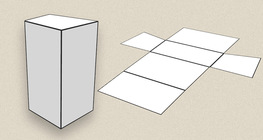#### Prisms

This animation demonstrates several types of prisms, from general to regular.

Informācija Ratio of volumes of similar solids#### Ratio of volumes of similar solids

This 3D scene explains the correlation between the ratio of similarity and the ratio of volume of geometric solids.

Informācija Volume and surface area (exercise)#### Volume and surface area (exercise)

An exercise about the volume and surface area of solids generated from a ´base cube´.

Informācija Dice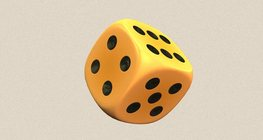#### Dice

Regular dice can be used for solving statistical and probability exercises.